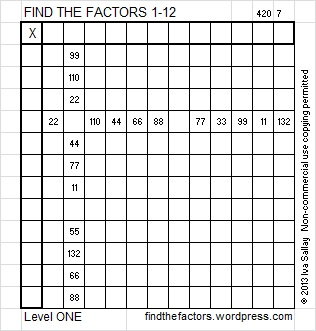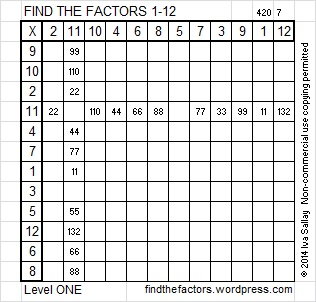# 122 and Level 1

122 is a composite number. 122 = 1 x 122 or 2 x 61. Factors of 122: 1, 2, 61, 122. Prime factorization: 122 = 2 x 61.122 is never a factor in the FIND THE FACTORS puzzles.

122 is the sum of two squares:
11² + 1² = 122

122 is the hypotenuse of a Pythagorean triple:
22-120-122 calculated from 2(11)(1), 11² – 1², 11² + 1²Excel file of this week’s puzzles and last week’s factors: 12 Factors 2014-05-19